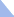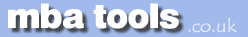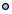MarketingFinanceOperations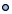EconomicsStatisticsStrategyMeetingsToolboxCommunicationmbatools.co.ukcompiled by

gari jenkins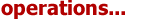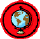Complex projects require a series of activities, some of which must be performed sequentially and others that can be performed in parallel with other activities. This collection of series and parallel tasks can be modelled as a network.

In 1957 the Critical Path Method (CPM) was developed as a network model for project management. CPM is a deterministic method that uses a fixed time estimate for each activity. While CPM is easy to understand and use, it does not consider the time variations that can have a great impact on the completion time of a complex project.

The Program Evaluation and Review Technique (PERT) is a network model that allows for randomness in activity completion times. PERT was developed in the late 1950's for the U.S. Navy's Polaris project having thousands of contractors. It has the potential to reduce both the time and cost required to complete a project.

The Network Diagram

In a project, an activity is a task that must be performed and an event is a milestone marking the completion of one or more activities. Before an activity can begin, all of its predecessor activities must be completed. Project network models represent activities and milestones by arcs and nodes. PERT originally was an activity on arc network, in which the activities are represented on the lines and milestones on the nodes. Over time, some people began to use PERT as an activity on node network. For this discussion, we will use the original form of activity on arc.

The PERT chart may have multiple pages with many sub-tasks. The following is a very simple example of a PERT diagram:

#### PERT Chart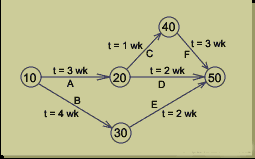The milestones generally are numbered so that the ending node of an activity has a higher number than the beginning node. Incrementing the numbers by 10 allows for new ones to be inserted without modifying the numbering of the entire diagram. The activities in the above diagram are labelled with letters along with the expected time required to complete the activity.

Steps in the PERT Planning Process

PERT planning involves the following steps:

1. Identify the specific activities and milestones.
2. Determine the proper sequence of the activities.
3. Construct a network diagram.
4. Estimate the time required for each activity.
5. Determine the critical path.
6. Update the PERT chart as the project progresses.

1.  Identify Activities and Milestones

The activities are the tasks required to complete the project. The milestones are the events marking the beginning and end of one or more activities. It is helpful to list the tasks in a table that in later steps can be expanded to include information on sequence and duration.

2.  Determine Activity Sequence

This step may be combined with the activity identification step since the activity sequence is evident for some tasks. Other tasks may require more analysis to determine the exact order in which they must be performed.

3.  Construct the Network Diagram

Using the activity sequence information, a network diagram can be drawn showing the sequence of the serial and parallel activities. For the original activity-on-arc model, the activities are depicted by arrowed lines and milestones are depicted by circles or "bubbles".

If done manually, several drafts may be required to correctly portray the relationships among activities. Software packages simplify this step by automatically converting tabular activity information into a network diagram.

4.  Estimate Activity Times

Weeks are a commonly used unit of time for activity completion, but any consistent unit of time can be used.

A distinguishing feature of PERT is it's ability to deal with uncertainty in activity completion times. For each activity, the model usually includes three time estimates:

• Optimistic time - generally the shortest time in which the activity can be completed. It is common practice to specify optimistic times to be three standard deviations from the mean so that there is approximately a 1% chance that the activity will be completed within the optimistic time.

• Most likely time - the completion time having the highest probability. Note that this time is different from the expected time.

• Pessimistic time - the longest time that an activity might require. Three standard deviations from the mean is commonly used for the pessimistic time.

PERT assumes a beta probability distribution for the time estimates. For a beta distribution, the expected time for each activity can be approximated using the following weighted average:

Expected time  =  ( Optimistic  +  4 x Most likely  +  Pessimistic ) / 6

This expected time may be displayed on the network diagram.

To calculate the variance for each activity completion time, if three standard deviation times were selected for the optimistic and pessimistic times, then there are six standard deviations between them, so the variance is given by:

[ ( Pessimistic  -  Optimistic ) / 6 ]2

5.  Determine the Critical Path

The critical path is determined by adding the times for the activities in each sequence and determining the longest path in the project. The critical path determines the total calendar time required for the project. If activities outside the critical path speed up or slow down (within limits), the total project time does not change. The amount of time that a non-critical path activity can be delayed without delaying the project is referred to as slack time.

If the critical path is not immediately obvious, it may be helpful to determine the following four quantities for each activity:

• ES - Earliest Start time
• EF - Earliest Finish time
• LS - Latest Start time
• LF - Latest Finish time

These times are calculated using the expected time for the relevant activities. The earliest start and finish times of each activity are determined by working forward through the network and determining the earliest time at which an activity can start and finish considering its predecessor activities. The latest start and finish times are the latest times that an activity can start and finish without delaying the project. LS and LF are found by working backward through the network. The difference in the latest and earliest finish of each activity is that activity's slack. The critical path then is the path through the network in which none of the activities have slack.

The variance in the project completion time can be calculated by summing the variances in the completion times of the activities in the critical path. Given this variance, one can calculate the probability that the project will be completed by a certain date assuming a normal probability distribution for the critical path. The normal distribution assumption holds if the number of activities in the path is large enough for the central limit theorem to be applied.

Since the critical path determines the completion date of the project, the project can be accelerated by adding the resources required to decrease the time for the activities in the critical path. Such a shortening of the project sometimes is referred to as project crashing.

6.  Update as Project Progresses

Make adjustments in the PERT chart as the project progresses. As the project unfolds, the estimated times can be replaced with actual times. In cases where there are delays, additional resources may be needed to stay on schedule and the PERT chart may be modified to reflect the new situation.

Benefits of PERT

PERT is useful because it provides the following information:

• Expected project completion time.

• Probability of completion before a specified date.

• The critical path activities that directly impact the completion time.

• The activities that have slack time and that can lend resources to critical path activities.

• Activity start and end dates.

Limitations

The following are some of PERT's weaknesses:

• The activity time estimates are somewhat subjective and depend on judgement. In cases where there is little experience in performing an activity, the numbers may be only a guess. In other cases, if the person or group performing the activity estimates the time there may be bias in the estimate.

• Even if the activity times are well-estimated, PERT assumes a beta distribution for these time estimates, but the actual distribution may be different.

• Even if the beta distribution assumption holds, PERT assumes that the probability distribution of the project completion time is the same as the that of the critical path. Because other paths can become the critical path if their associated activities are delayed, PERT consistently underestimates the expected project completion time.

The underestimation of the project completion time due to alternate paths becoming critical is perhaps the most serious of these issues. To overcome this limitation, Monte Carlo simulations can be performed on the network to eliminate this optimistic bias in the expected project completion time.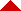Operations Project Management Revision Sheets Articles